# A History of Fractal Geometry

Any mathematical concept now well-known to school children has gone through decades, if not centuries of refinement. A typical student will, at various points in her mathematical career -- however long or brief that may be -- encounter the concepts of dimension, complex numbers, and "geometry". If the field of mathematics does not particularly interest her, this student might see these concepts as distinct and unrelated and, in particular, she might make the mistake of thinking that the Euclidean geometry taught to her in school encompasses the whole of the field of geometry. However, if she were to pursue mathematics at the university level, she might discover an exciting and relatively new field of study that links the aforementioned ideas in addition to many others: fractal geometry.

While the lion's share of the credit for the development of fractal geometry goes to Benoît Mandelbrot, many other mathematicians in the century preceding him had laid the foundations for his work. Moreover, Mandelbrot owes a great deal of his advancements to his ability to use computer technology -- an advantage that his predecessors distinctly lacked; however, this in no way detracts from his visionary achievements. Nevertheless, while acknowledging and understanding the accomplishments of Mandelbrot, it undoubtedly helps to have some familiarity with the relevant works of Karl Weierstrass, Georg Cantor, Felix Hausdorff, Gaston Julia, Pierre Fatou and Paul Lévy -- not only to make Mandelbrot's work clearer -- but to see its connections to other branches of mathematics. Equally, while most authors will not fail to include at least brief discussion of Mandelbrot's rather interesting and slightly unconventional (for a modern mathematician) life in their texts on fractals, it seems only fair to give some, if not equal, consideration to his predecessors.

Until the 19th century, mathematics had concerned itself only with functions that produced differentiable curves. Indeed, the conventional wisdom of the day said that any function with an analytic formula (i.e. sum of a convergent power series) would certainly produce such a curve.  However, on July 18, 1872, Karl Weierstrass presented a paper at the Royal Prussian Academy of Sciences showing that for $a$ a positive integer and $0 < b < 1$
$\sum_{n=1}^{\infty} b^{n}\cos(a^{n}x \pi)$
is not differentiable. Using the limit definition of a derivative, he showed that the difference quotient of the function
$[f (x + h) - f (x)]/h$
gets arbitrarily large as the index of summation increases.
As Weierstrass himself pointed out, Riemann had introduced
$\sum_{n=1}^{\infty} \sin(n^{2}x)/n^{2}$
as an example of a non-differentiable analytic function, but never published a proof, nor could anyone replicate it.  Thus, Weierstrass's proof stands as the first rigorously proven example of a function that is analytic, but not differentiable. While Weierstrass, and indeed, much of the mathematical establishment of the time eschewed the use of graphs in favour of symbolic manipulation in order to prove results, future mathematicians such as Helge von Koch and Mandelbrot himself found it useful to represent their results graphically.   Indeed, when one has only worked with curves that are differentiable almost everywhere, an obvious question when one encounters a formula for a curve that is not is, "what does it look like?"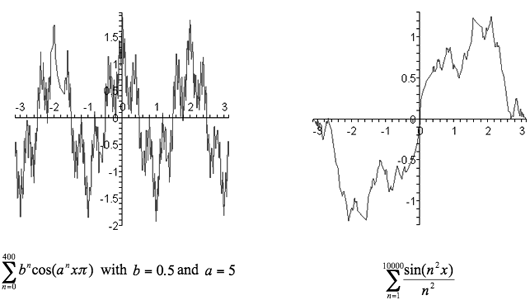While these are both approximations, one can see that these functions lack the smoothness of parabolas or of the sine and cosine functions. These functions resisted traditional analysis and were -- though not due to their appearance, which was beyond the ability of mathematicians of the day to represent -- labelled "monsters" by Charles Hermite and were largely ignored by the contemporary mathematical community. 

In 1883 Georg Cantor, who attended lectures by Weierstrass during his time as a student at the University of Berlin  and who is to set theory what Mandelbrot is to fractal geometry,  introduced a new function, ψ , for which ψ' = 0 except on the set of points, $\{z\}$. This set, $\{z\}$, is what became known as the Cantor set.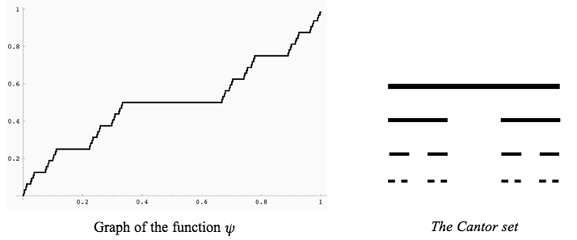The function ψ is singular, monotone, non-constant and ψ' = 0 almost everywhere. It also has the property that
ψ(1) - ψ(0) = 1 however $\int_{0}^{1} \psi' (x) dx = 0$ 
The Cantor set has a Lebesgue measure of zero; however, it is also uncountably infinite.  What is more, it has the property of being self-similar, meaning that if one magnifies a section of the set, one obtains the whole set again. Looking at Figure 4, one can easily see that each horizontal line is one third the size of the horizontal line directly above it. In fact, self-similarity is a feature of fractals, and the Cantor set is an early example of a fractal, though self-similarity was not defined until 1905 (by Cesàro, who was analysing the paper by Helge von Koch discussed below) and fractals were not defined until Mandelbrot in 1975,  thus Cantor would not have thought of it in those terms.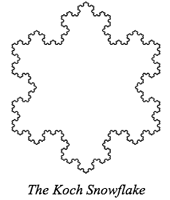In a paper published in 1904, Swedish mathematician Helge von Koch constructed using geometrical means the now-famous von Koch curve and hence the Koch snowflake, which is three von Koch curves joined together. In the introduction to his paper he stated the following about Weierstrass's 1872 essay :
... it seems to me that his [Weierstrass's] example is not satisfactory from the geometrical point of view since the function is defined by an analytic expression that hides the geometrical nature of the corresponding curve and so from this point of view one does not see why the curve has no tangent. Rather it seems that the appearance is actually in contradiction with the factual reality established by Weierstrass in a purely analytic way.
Von Koch's curve, like the Cantor set, has the property of self-similarity. It, too, is a fractal, though, like Cantor, von Koch was not thinking in such terms. He merely aimed to provide an alternative way of proving that functions that were non-differentiable (i.e. functions that "have no tangents" in geometric parlance) could exist -- a way that involved using "elementary geometry" (reference 's title translates to On a Continuous Curve without Tangent Constructible from Elementary Geometry). In doing so, von Koch expressed a link between these non-differentiable "monsters" of analysis and geometry.

Von Koch himself was a fairly unremarkable mathematician. Many of his other results were derived from those of Henri Poincaré, from whom he knew it was possible to obtain "pathological" results -- i.e. these so-called "monsters" -- but never really explored them, outside of the aforementioned essay.  Poincaré, it should be noted, studied non-linear dynamics in the later 19th century, which eventually led to chaos theory,  a field closely related to fractal geometry, though beyond the scope of this paper. It is therefore fitting that a mathematician whose work followed that of Poincaré so closely would turn out to be one of the forefathers of a field that is closely related to the area of study for which Poincaré himself helped lay the foundations.

An absolutely key concept in the study of fractals, aside from the aforementioned self-similarity and non-differentiability, is that of Hausdorff dimension, a concept introduced by Felix Hausdorff in March of 1918. Hausdorff's results from the same paper were important to the field of topology, as well;  however that his definition of dimension extended the previous definition to allow for sets to have a dimension that is an arbitrary, non-zero value  (unlike topological dimension) ended up being integral to the definition of a fractal, as Mandelbrot defined fractals "a set having Hausdorff dimension strictly greater than its topological dimension." 

As soon as Hausdorff introduced this new, expanded definition of dimension, it was the subject of investigation -- in particular by Abraham Samilovitch Besicovitch, who, from 1934 to early 1937 wrote no less than three papers referencing Hausdorff's work.  Sadly, by this time, Hausdorff was experiencing difficulties living as a Jew in Nazi Germany. He was forced to give up his post as a professor at the University of Bonn in 1935, and even though he continued to work on set theory and topology, his work could only be published outside of Germany. Despite temporarily managing to avoid being sent to a concentration camp, the situation in Germany quickly became unbearable and, with nowhere else to go, he, along with his wife and sister-in-law, opted to commit suicide in January 1942. 

The Hausdorff dimension, $d$, of a self-similar set -- its connection to fractal geometry, though, as previously stated, there are many other applications of Hausdorff dimension -- which is scaled down by ratios $r_{1} , r_{2} , ... , r_{N}$ (i.e. the first iteration of the set is the whole set, scaled down by a factor of $r_{1}$) satisfies the following two equations :
$r_{1}^{d} + r_{2}^{d} + ... + r_{N}^{d} = 1$ and $Nr^{d} =1$.
These equations, however, do not appear in Hausdorff's paper, as they relate directly to fractals (and calculating the dimension of a fractal), which were ideas that would have been unknown to Hausdorff. Still, from these two equations, it is easy to see how one can obtain a dimension that is not a whole number, as 
$d = \Large \frac{\log(N)}{ \log(\log(1/r))}$.
At nearly the same time that Hausdorff did his research, two French mathematicians, Gaston Julia and Pierre Fatou, developed results (though not together) that ended up being important to fractal geometry. They studied mappings of the complex plane and iterative functions. Their work with iterative functions led to the ideas of attractors, points in space which attract other points to them; and repellors, points in space that repel other points, usually to another attractor. These concepts are also important to chaos theory. The boundaries of the various basins of attraction turned out to be very complicated and are known today as Julia sets,  an example of which can be seen in Figure 6. A more analytic definition of a Julia set for a function, $f (z)$, is 
$J (f ) = \partial \{z | f ^{(n)}(z) \to ∞$ as $n \to \infty \}$.
Namely, "the Julia set of $f$ is the boundary of the set of points $z \in \mathbb{C}$ that escape to infinity under repeated iteration by $f (z)$."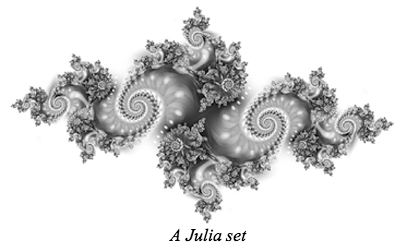Because Fatou and Julia (and, by extension, their work) predated computers, they were unable to generate pictures such as the one on the right, which is the graph of millions of iterations of a function. They were limited to what they could do by hand, which would only be about three or four iterations.  Julia published a 199-page paper in 1918 called Mémoire sur l'iteration des fonctions rationelles , which discussed much of his work on iterative functions and describing the Julia set. With this paper, Julia won the Grand Prix of the Académie des Sciences and became extremely famous in mathematical circles throughout the 1920s. However, despite this prominence, his work on iteration fell into obscurity for about fifty years. 

Fatou, on the other hand, did not achieve the same level of fame as Julia, even contemporarily, despite discovering very similar results -- though in a different manner -- and also submitting them to be published. He submitted an announcement of his results to Comptes Rendus, while Julia had chosen to send his opus to the Journal de Mathématiques Pures et Appliquées. Julia, protective of his work, sent letters to Comptes Rendus asking them to investigate whose results had priority. The publication duly launched an investigation and included a note on Julia's findings in the same issue as the Fatou's announcement. This apparently discouraged Fatou enough to keep him from entering for the Grand Prix. Still, the Académie des Sciences gave him some recognition and awarded him a prize for his paper on the topic. 

Julia sets can be completely disconnected, in which case they are "dust" (Figure 7) -- similar to the Cantor set (Figure 4) -- or they are completely connected (Figure 6). On rare occasions, they can be "dendrites" (Figure 8), where they are "made up completely of continuously sub-branching lines, which are only just connected since the removal of any point from them would split them in two,"  at which point, they would be considered "dust".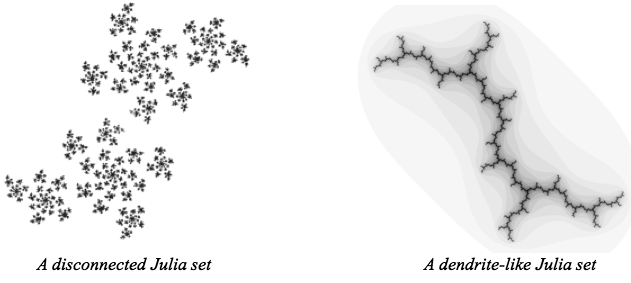The method for deciding whether or not a set is connected is to calculate out the orbit of the starting point. The orbit for a starting point, $x_{0}$ , is the sequence 
$(x_{0} , x_{1} , x_{2} , ... )$ where for each $i \in \mathbb{N}$ we have $x_{i} = f (x_{i-1})$.
If this sequence goes off to infinity, then the set is disconnected. Otherwise, it is connected.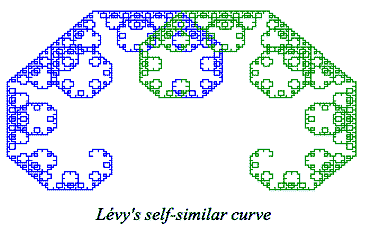In 1938, the year after Besicovitch's last paper on Hausdorff dimension, Paul Lévy produced a comprehensive treatment on the property of self-similarity. He showed that the von Koch curve was just one of many examples of a self-similar curve, though von Koch himself had stated that his curve could be generalized. The curves generated by Lévy (see Figure 9 for an example -- the green and blue sets are two smaller copies of the larger set) were iterative and connected and, with enough iterations, covers (or tiles) the plane. Lévy's curves, however, are not fractals, as they have both a Hausdorff and a topological dimension of two. 

Little did anyone at this time suspect that there was someone, albeit still a very young person, who would unite the works of Lévy and Hausdorff. Benoit Mandelbrot was born in 1924 in Warsaw, Poland and, like Hausdorff, he was also Jewish, though his family managed to escape life under the Third Reich in 1936 by leaving Poland for France, where family and friends helped them set up their new lives. One of Mandelbrot's uncles, Szolem Mandelbrojt, was a pure mathematician, who took an interest in the young Mandelbrot and tried to steer him towards mathematics. In fact, in 1945, Mandelbrojt showed his nephew the works of Fatou and Julia, though the young Mandelbrot initially did not take much of an interest. 

Mandelbrot's education was very uneven, and completely interrupted in 1940, when Mandelbrot and his family were forced to flee the Nazis again. This time they went to central France. Mandelbrot, like Helge von Koch before him, preferred visual representations of mathematical problems, as opposed to the symbolic,  though this may also stem from his lack of formal education, due to World War II.  Unfortunately, this would bring him into direct conflict with the teaching style of "Bourbaki", a group of mathematicians whose belief in solving problems analytically (as opposed to visually) dominated the teaching of mathematics in France at the time. 

After the war had ended, Mandelbrot took the entrance exams for the École Polytechnique in Paris, despite having no preparation. He did very well in the mathematics section, where he could employ his ability to solve problems through visualisation to answer questions. While this method was not always possible on other sections, he managed to pass  and after a one-day career at the École Normale, Mandelbrot started at the École Polytechnique, where he met another of his mentors, Paul Lévy,  who was a professor at there from 1920 until his retirement in 1959 .

After completing his studies, Mandelbrot moved to New York, where he started work for IBM's Thomas J. Watson Research Centre. The company gave him a free hand in choosing a topic of study, which allowed him to explore and develop concepts using his own methods, without having to worry about the reaction of the academic community. In 1967, while still there, Mandelbrot wrote his landmark essay, How Long Is the Coast of Britain? Statistical Self-Similarity and Fractional Dimension , in which he linked the idea of previous mathematicians to the real world -- namely coastlines, which he claimed were "statistically self-similar". He argued that 
Self-similarity methods are a potent tool in the study of chance phenomena, including geostatics, as well as economics and physics. In fact, many noises have dimensions D contained between 0 and 1 ...
After this essay and with the aid of computers, Mandelbrot returned to the work of Julia and Fatou. With the ability to see, for the first time, what these sets looked like in their limits, Mandelbrot came up with the idea of mapping the values of $c \in \mathbb{C}$ for which the Julia set for the function $f_{c} (z) = z^{2} + c$ is connected. This creates the Mandelbrot set, $M$ (Figure 10), which is more formally denoted as
$M = \{c \in \mathbb{C} | f_{c}^{(n)}(z)$ is finite as $n \to \infty$}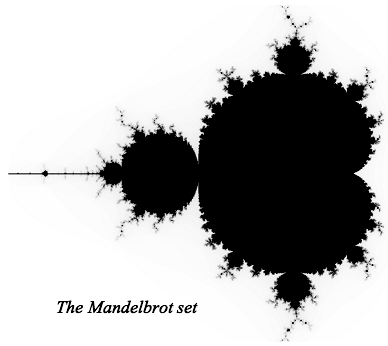The Mandelbrot set is, for many, the quintessential fractal. When one zooms in on some part of the edge, one notices that the Mandelbrot set is, indeed, self-similar. Furthermore, if one zooms in even further on various sections of the edge, one obtains different Julia sets. In fact, it is "asymptotically similar to Julia sets near any point on its boundary," as proved in a theorem by the Chinese mathematician Tan Lei. 

Mandelbrot has managed not only to invent the discipline of fractal geometry, but has also popularized it through its applications to other areas of science. He clearly believed this was important, as he once stated 
The rare scholars who are nomads-by-choice are essential to the intellectual welfare of the settled disciplines.
As he hinted in How Long Is the Coast of Britain? fractal geometry comes in useful in representing natural phenomena; things such as coastlines, the silhouette of a tree, or the shape of snowflakes -- things are not easily represented using traditional Euclidean geometry. After all, no organic entity comes to mind when one contemplates a square or a circle. Equally, no simple shape from Euclidean geometry comes to mind when contemplating things such as the path of a river. Even the earth is not a perfect sphere, however convenient it may be for one's calculations to treat it as such. Furthermore, fractal geometry and chaos theory have important connections to physics, medicine, and the study of population dynamics.  However, even if the field lacked these links, it would be hard for those so inclined to resist the aesthetic appeal of most fractals.

Mandelbrot's non-traditional approach led him to invent an amazing and useful new form of mathematics. However, no mathematician can claim to have developed his results in complete isolation from anyone else's. Mandelbrot's discovery owes a great deal to the mathematicians who preceded him, such as Weierstrass and von Koch, but especially to Julia, Fatou, and Hausdorff. He also benefitted from access to computers, which allowed him not only to build upon the works of others in a new way -- one which had definitely not been done before -- but to use his preferred method of solving problems -- namely visualisation. Furthermore, his invention also makes a case for the importance of the study of pure mathematics: until Mandelbrot came along and united the eclectic ideas of Hausdorff, Julia, et al, they represented very abstract mathematical ideas from varying branches of (pure) mathematics. There is very little that would interest an ordinary biologist about set theory. However, through fractal geometry, many of these seemingly abstract ideas (from mathematicians who are relatively unknown outside of their own spheres of research) develop applications that other scientists and even non-scientists can appreciate. Thus, the work that eventually led to fractals and their applications are an excellent counterexample to the arguments of anyone who would dare to denigrate the study of pure mathematics.

### References (show)

1. G Cantor, De la puissance des ensembles parfait de points. 1884. Classics on Fractals. Ed. A E Gerald ( Addison -Wesley, 1993).
2. R M Crownover, Introduction to Fractals and Chaos (London, 1995).
3. G A Edgar, ed. Classics on Fractals (Addison-Wesley, 1993).
4. F Hausdorff, Dictionary of Scientific Biography. 1972
5. H von Koch, Dictionary of Scientific Biography. 1972
6. H vonKoch, Sur une courbe continue sans tangente obtenue par une construction géométrique élémentaire. 1904. Classics on Fractals . Ed. A E Gerald (Addison -Wesley, 1993).
7. N Lesmoir-Gordon, W Rood, and R Edney. Introducing Fractal Geometry (Cambridge, 2000).
8. B Mandelbrot, How Long Is the Coast of Britain? Statistical Self-Similarity and Fractional Dimension. Science, New Series 156 3775 (May 5, 1967): 636-638.
9. J J O'Connor, J.J., and E F Robertson. MacTutor: Cantor biography
10. ---. MacTutor: Fatou biography.
11. ---. MacTutor: Julia biography.
12. ---. MacTutor: Levy, Paul biography.
13. ---. MacTutor: Mandelbrot biography.
14. K Weierstrass, Über continuirliche Functionen eines reellen Arguments, die für keinen Werth des Letzteren einen bestimmten Differentialquotienten besitzen. 1872. Classics on Fractals . Ed. A E Gerald (Addison -Wesley, 1993).

Written by Holly Trochet (University of St Andrews)
Last Update February 2009